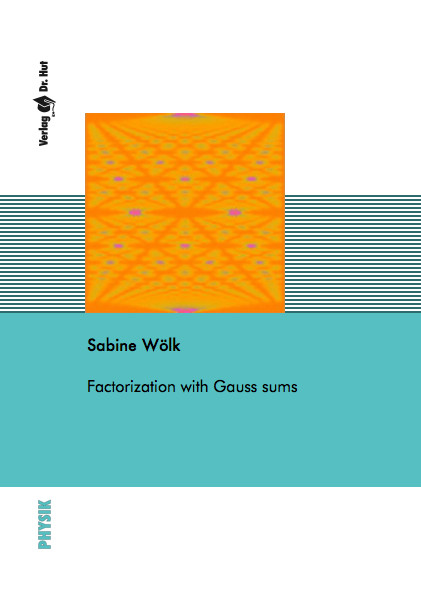Datenbestand vom 20. August 2019aktualisiert am 20. August 2019

# ISBN 9783843900263Euro 96,00 inkl. 7% MwSt

978-3-8439-0026-3, Reihe Physik

Sabine Wölk
Factorization with Gauss sums

127 Seiten, Dissertation Universität Ulm (2011), Hardcover, A5

## Zusammenfassung / Abstract

In summary, this thesis continues the work of W. Merkel by further investigations of the factorization abilities of Gauss sums. Furthermore, it investigates the similarities and differences of Shor’s algorithm compared to Gauss sum factorization, which leads us to a deeper understanding of both algorithms. Although, we have outlined a possibility for a fast Gauss sum factorization algorithm there is still the problem of information being encoded in the zeros of the probability distribution.

In this thesis, we have pointed out strong connection between the standard Gauss sum and the reciprocate Gauss sum. These connection suggested, that it might by also possible to factorize with a truncated standard Gauss sum.

Furthermore, we need to find an algorithm which extracts information efficiently out of the zeros of a probability distribution. In qbit systems it might be useful to transform every single qubit by a not gate and then analyze the autocorrelation function between the initial state and the transformed state.